The MI Procedure

Monotone Methods for Data Sets with Monotone Missing Patterns

For data sets with monotone missing data patterns, you can use monotone methods to impute missing values for the variables. A monotone method creates multiple imputations by imputing missing values sequentially over the variables taken one at a time.

For example, with variables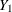,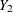, …,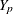(in that order) in the VAR statement, a monotone method sequentially simulates a draw for missing values for variables, …,. That is, the missing values are imputed by using the sequence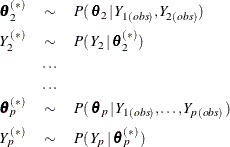where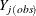is the set of observed
values,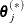is the set of simulated parameters for the conditional distribution ofgiven covariates constructed from variables,, …,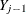, and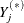is the set of imputedvalues.

The missing values for the leading variableare not imputed, and missing values for, …,are not imputed for those observations with missingvalues. For each subsequent variablewith missing values, the corresponding imputation method is used to fit a model with covariates constructed from its preceding variables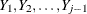. The observed observations for, which include only observations with observed values for, are used in the model fitting. With this resulting model, a new model is drawn and then used to impute missing values for.

You can specify a separate monotone method for each imputed variable. If a method is not specified for the variable, then the default method is used. That is, a regression method is used for a continuous variable and a discriminant function method is used for a classification variable. For each imputed variable, you can also specify a set of covariates that are constructed from its preceding variables. If a set of covariates is not specified for the variable, all preceding variables in the VAR list are used as covariates.

You can use a regression method, a predictive mean matching method, or a propensity score method to impute missing values for a continuous variable; a logistic regression method for a classification variable with a binary or ordinal response; and a discriminant function method for a classification variable with a binary or nominal response. See the sections Monotone and FCS Regression Methods, Monotone and FCS Predictive Mean Matching Methods, Monotone Propensity Score Method, Monotone and FCS Discriminant Function Methods, and Monotone and FCS Logistic Regression Methods for these methods.